Question

# p8-15 A-C 333 CHAPTER 8 Risk and Return a. If the returns of assets V and...

p8-15 A-C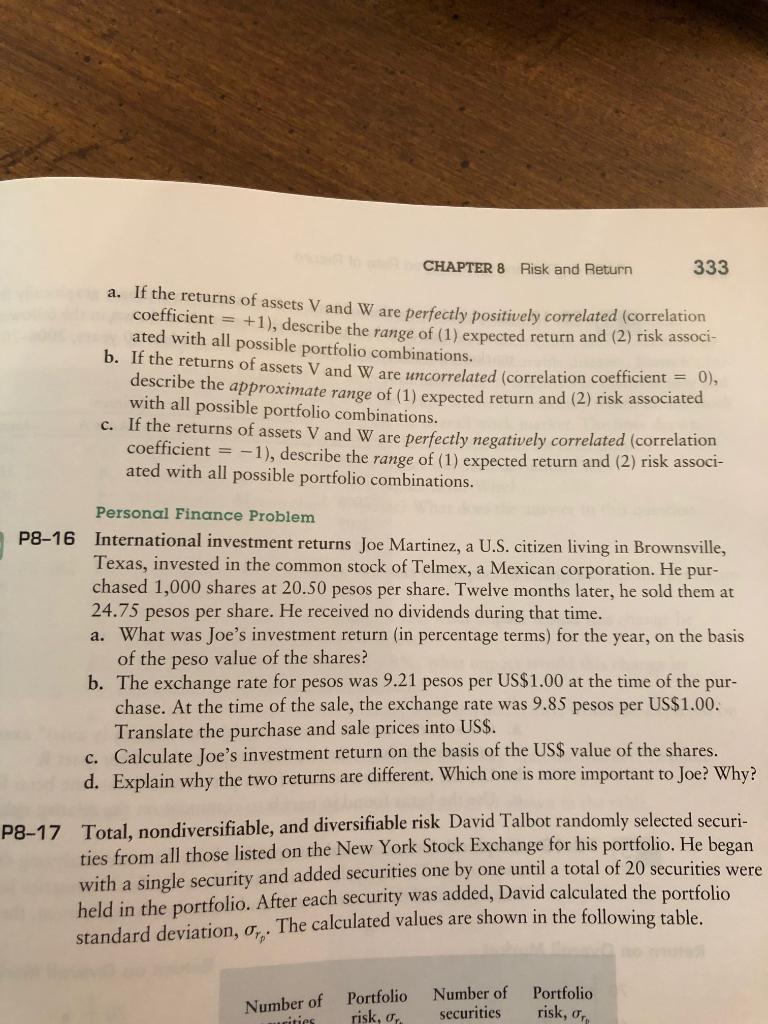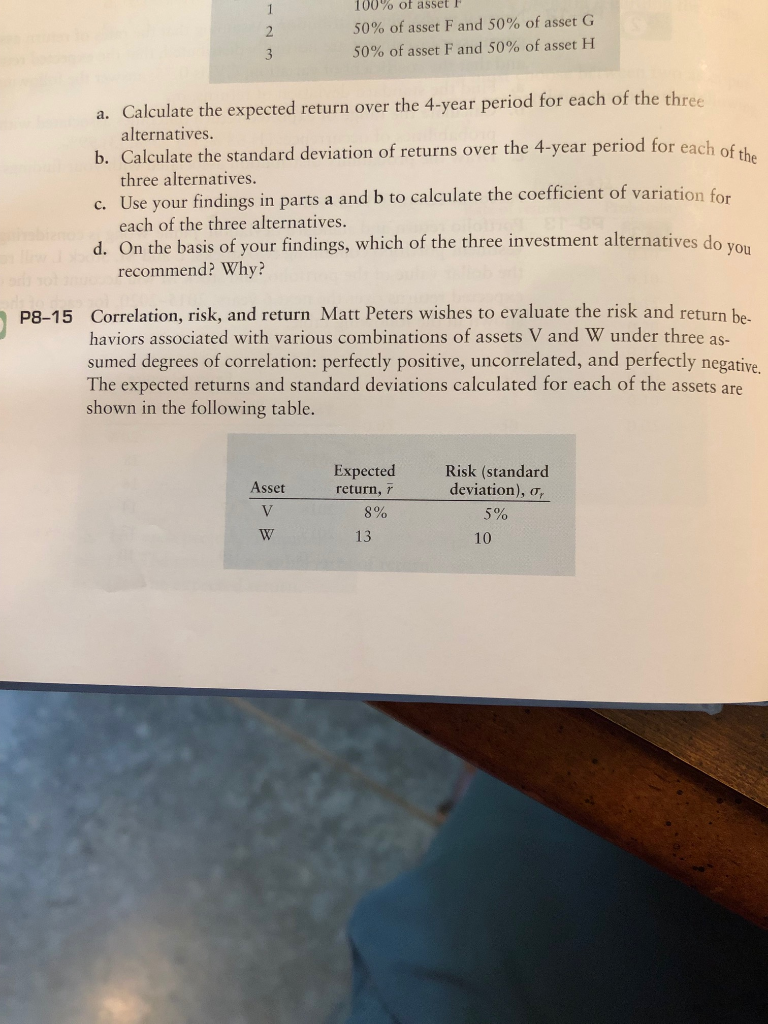333 CHAPTER 8 Risk and Return a. If the returns of assets V and W are perfectly positively correlated (correlation coefficient = +1), describe the range of (1) expected return and (2) risk associ- ated with all possible portfolio combinations. b. If the returns of assets V and W are uncorrelated (correlation coefficient = 0), describe the approximate range of (1) expected return and (2) risk associated with all possible portfolio combinations c. If the returns of assets V and W are perfectly negatively correlated (correlation coefficient -1), describe the range of (1) expected return and (2) risk associ- ated with all possible portfolio combinations. Personal Finance Problem P8-16 International investment returns Joe Martinez, a U.S. citizen living in Brownsville, Texas, invested in the common stock of Telmex, a Mexican corporation. He pur- chased 1,000 shares at 20.50 pesos per share. Twelve months later, he sold them at 24.75 pesos per share. He received no dividends during that time. a. What was Joe's investment return (in percentage terms) for the year, on the basis of the peso value of the shares? b. The exchange rate for pesos was 9.21 pesos per US\$1.00 at the time of the pur- chase. At the time of the sale, the exchange rate was 9.85 pesos per US\$1.00. Translate the purchase and sale prices into US\$. c. Calculate Joe's investment return on the basis of the US\$ value of the shares d. Explain why the two returns are different. Which one is more important to Joe? Why? P8-17 Total, nondiversifiable, and diversifiable risk David Talbot randomly selected securi- ties from all those listed on the New York Stock Exchange for his portfolio. He began with a single security and added securities one by one until a total of 20 securities were held in the portfolio. After each security was added, David calculated the portfolio standard deviation, o,. The calculated values are shown in the following table Number of securities Portfolio risk, o, Portfolio risk, a Number of ucities
100% of asset F 1 50% of asset F and 50% of asset G 2 50% of asset F and 50% of asset H 3 a. Calculate the expected return over the 4-year period for each of the three alternatives. b. Calculate the standard deviation of returns over the 4-year period for each of the three alternatives. c. Use your findings in parts a and b to calculate the coefficient of variation for each of the three alternatives. d. On the basis of your findings, which of the three investment alternatives do you recommend? Why? P8-15 Correlation, risk, and return Matt Peters wishes to evaluate the risk and return be haviors associated with various combinations of assets V and W under three as- sumed degrees of correlation: perfectly positive, uncorrelated, and perfectly negative The expected returns and standard deviations calculated for each of the assets are shown in the following table. Expected Risk (standard deviation), o, Asset return, r V 8% 5% 13 10

SEE THE IMAGE. ANY DOUBTS, FEEL FREE TO ASK. THUMBS UP PLEASE

CHANGE IN CORRELATION COEFFICIENT DOES NOT AFFECT RETURNS, SO EXPECTED RANGE OF RETURNS REMAIN SAME IN ALL 3 CASES.

THE RISK = STANDARD DEVIATION WILL BE LOWEST WHEN THE CORRELATION IS NEGATIVE, AS IT LEADS TO FAVOURABLE DIVERSIFICATION

I SOLVED THIS SUM EARLIER, IT WAS MENTIONED TO ROUND TO 1 DECIMAL SO I HAVE ROUNDED TO STANDARD DEVIATION = RISK TO 1 DECIMAL.

IF YOU WANT TO MAKE ME CHANGE, LET ME KNOW TILL WHAT DECIMALS, I SHOULD ROUND.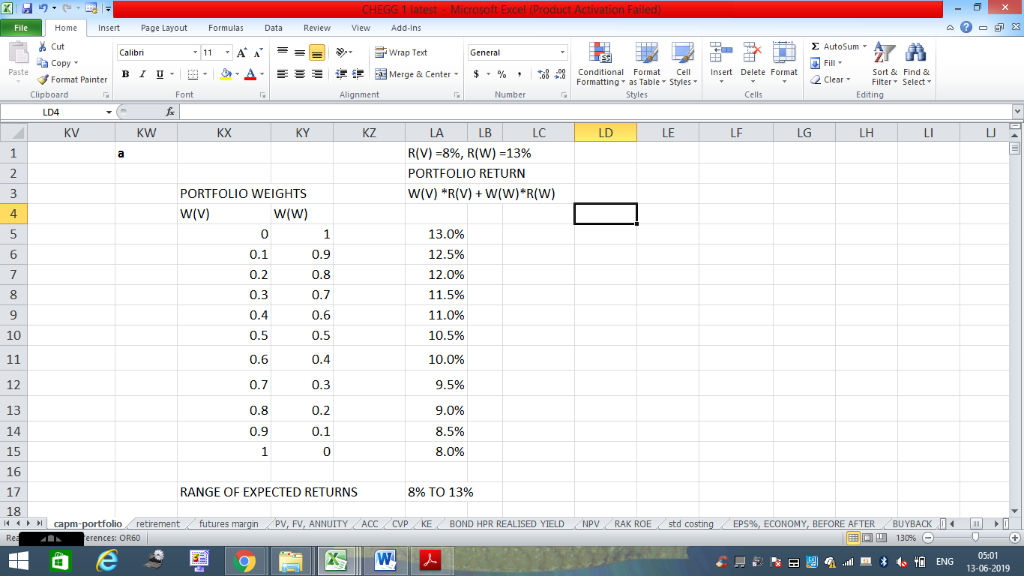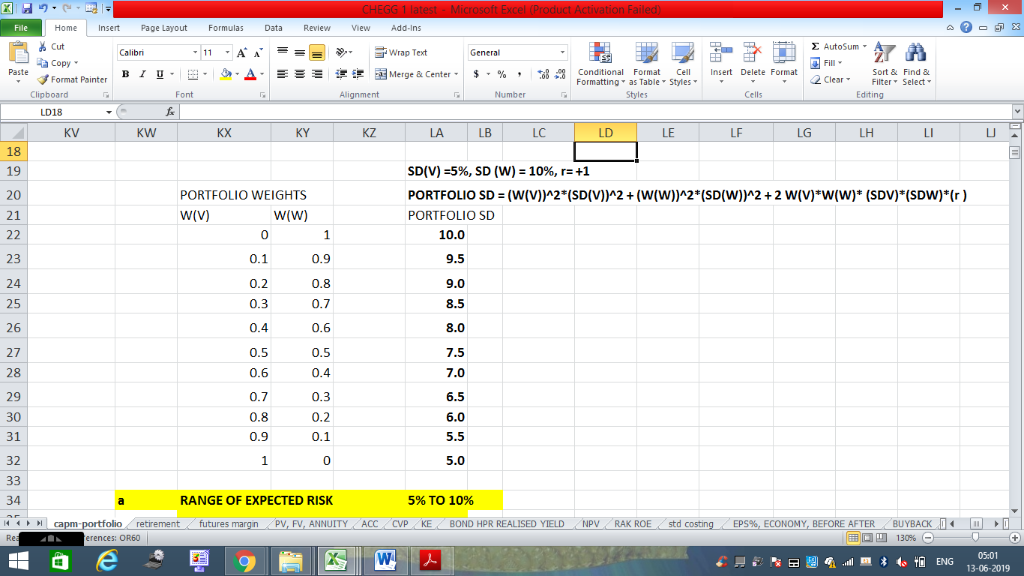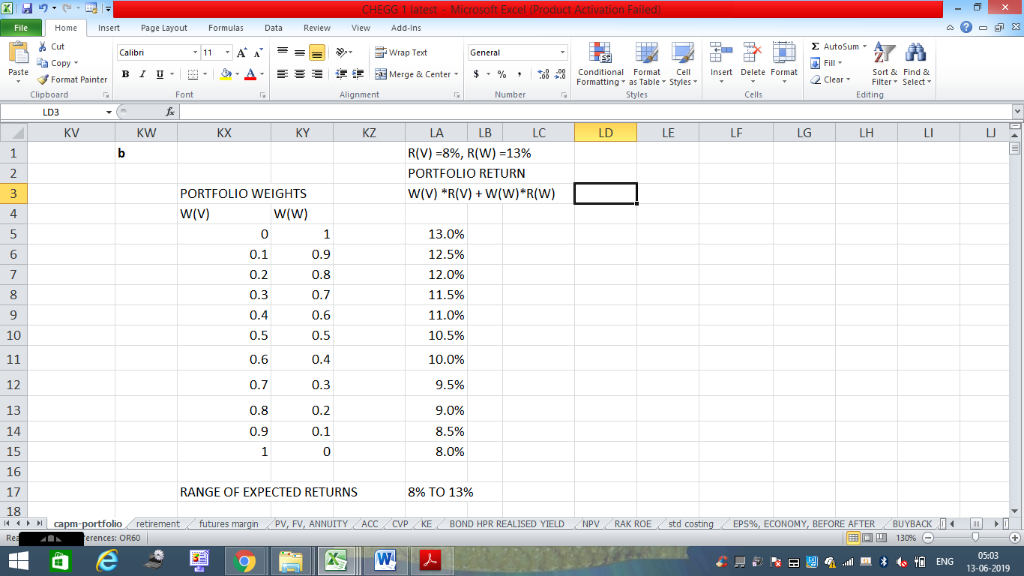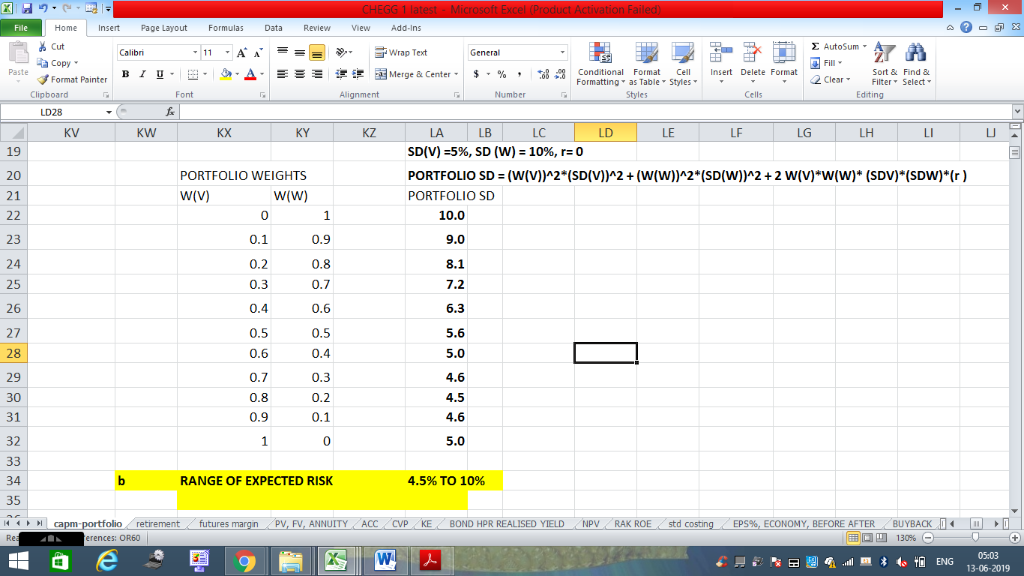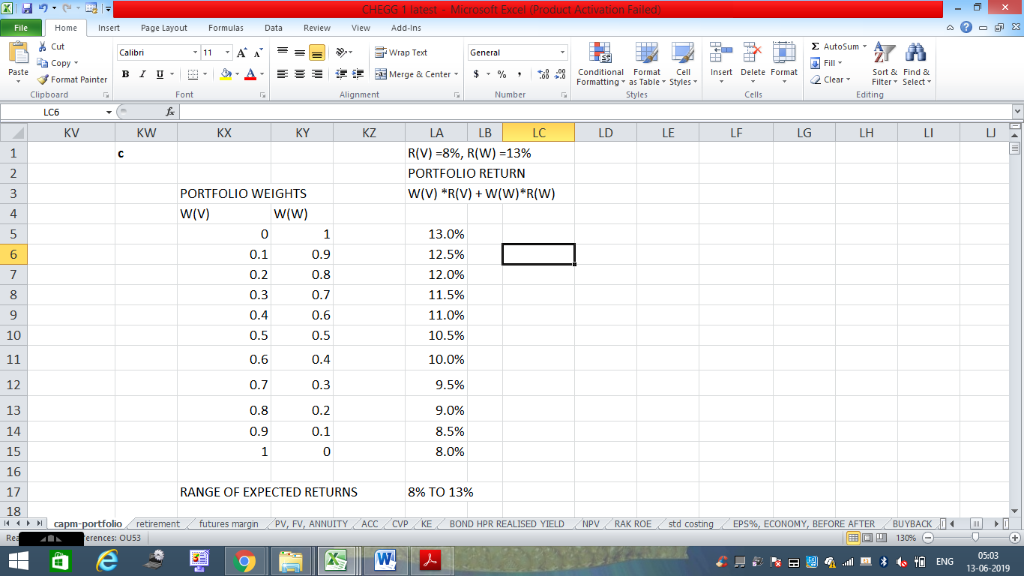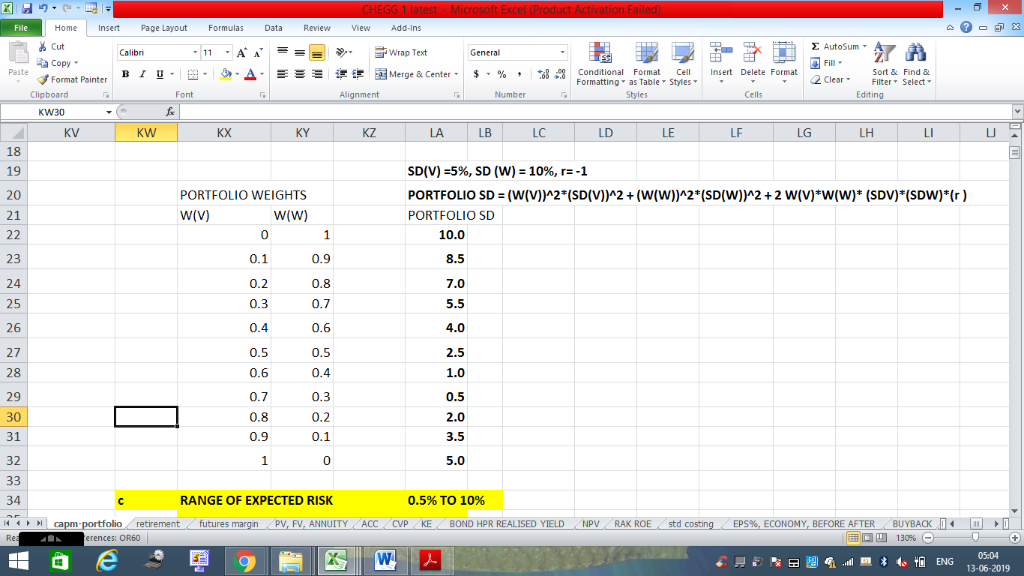#### Earn Coins

Coins can be redeemed for fabulous gifts.

Similar Homework Help Questions
• ### le three alternatives. c. Use your findings in parts a and b to calculate the coefficient of variatio each of the three...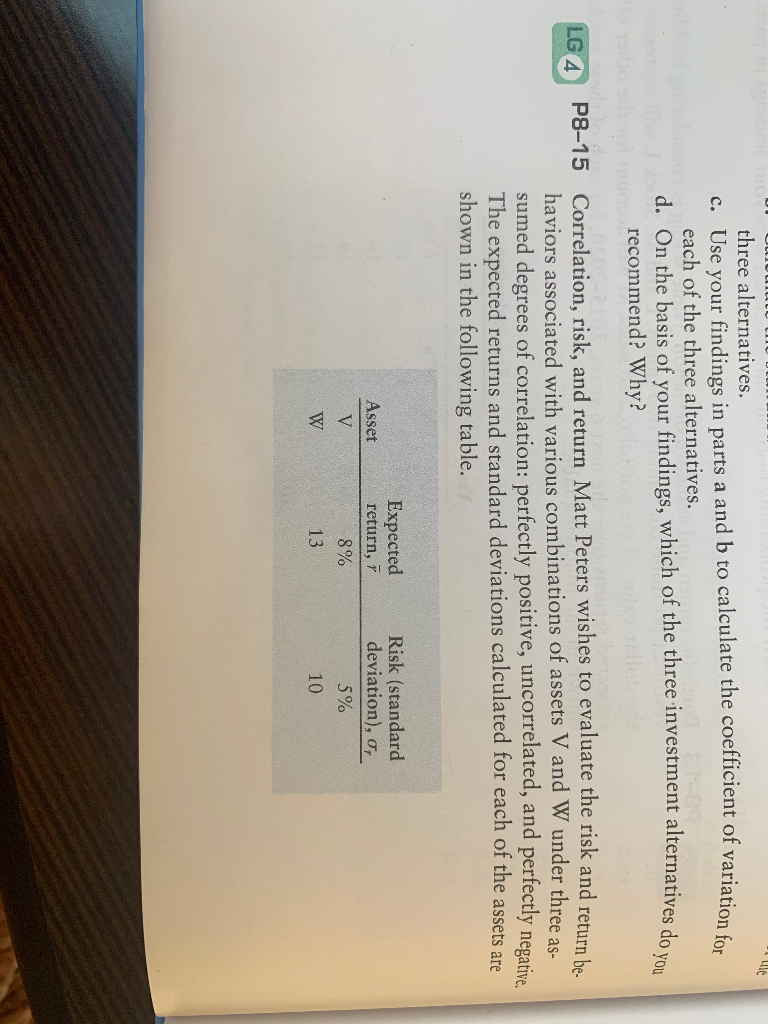le three alternatives. c. Use your findings in parts a and b to calculate the coefficient of variatio each of the three alternatives. d. On the basis of your findings, which of the three investment alternatives do recommend? Why? LG 4 P8-15 Correlation, risk, and return Matt Peters wishes to evaluate the risk and return be haviors associated with various combinations of assets V and W under three as- sumed degrees of correlation: perfectly positive, uncorrelated, and perfectly negative The...

• ### Assume you wish to evaluate the risk and return behaviors associated with various combinations of assets...

Assume you wish to evaluate the risk and return behaviors associated with various combinations of assets V and W under three assumed degrees of​ correlation: perfect​ positive, uncorrelated, and perfect negative. The following average return and risk values were calculated for these​ assets: Asset    Average​ Return, r Risk​ (Standard Deviation), s V 7.9​% 4.6​% W 12.7​% 9.7​% a. If the returns of assets V and W are perfectly positively correlated​ (correlation coefficient = + 1​), describe the range of​...

• ### LG4 5-14 Correlation, risk, and return Matt Peters wishes to evaluate the risk and return behaviors...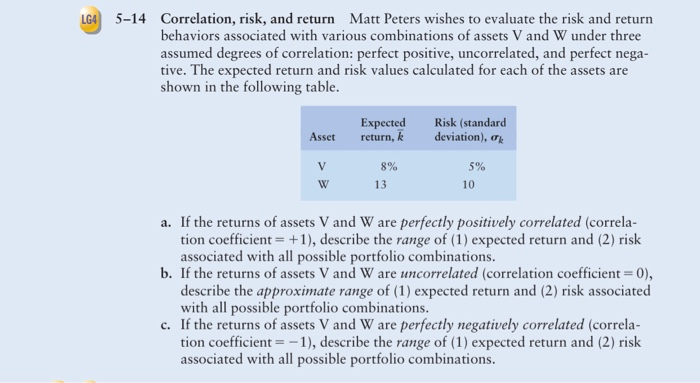LG4 5-14 Correlation, risk, and return Matt Peters wishes to evaluate the risk and return behaviors associated with various combinations of assets V and W under three assumed degrees of correlation: perfect positive, uncorrelated, and perfect nega- tive. The expected return and risk values calculated for each of the assets are shown in the following table. Asset Expected return, k Risk (standard deviation), V 5% 8% 13 a. If the returns of assets V and W are perfectly positively correlated...

• ### Correlation, risk, and return Matt Peters wishes to evaluate the risk and return behaviors associated with...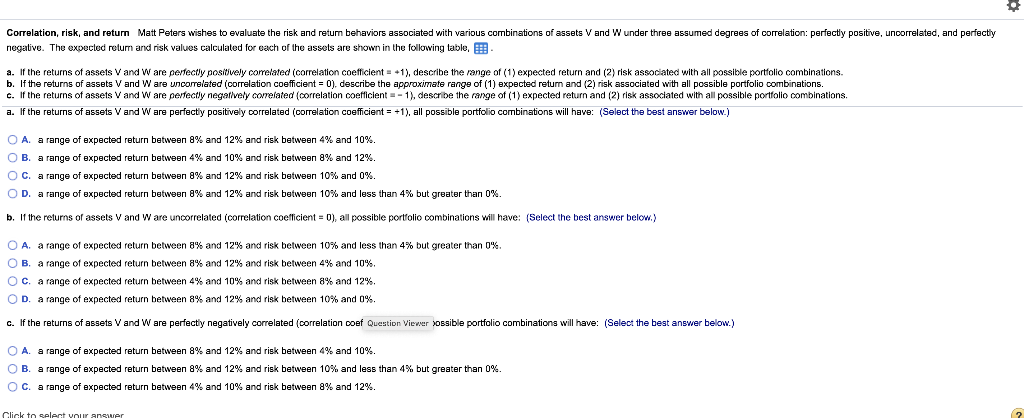Correlation, risk, and return Matt Peters wishes to evaluate the risk and return behaviors associated with various combinations of assets V and W under three assumed degrees of correlation: perfectly positive, uncorrelated, and perfectly negative. The expected return and risk values calculated for each of the assets are shown in the following table, B a. If the returns of assets V and W are perfectly positively correlated correlation coefficient = +1), describe the range of (1) expected return and (2)...

• ### P8-13 (similar to) Question Help Portfolio return and standard deviation Personal Finance Problem Jamie Wong is...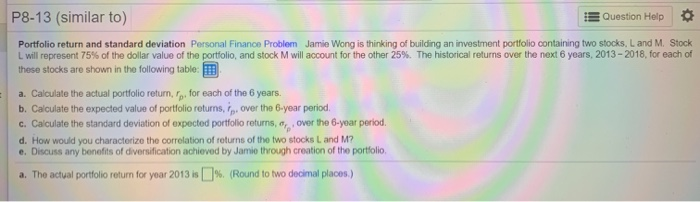P8-13 (similar to) Question Help Portfolio return and standard deviation Personal Finance Problem Jamie Wong is thinking of building an investment portfolio containing two stocks, L and M. Stock Lwill represent 75% of the dollar value of the portfolio, and stock M will account for the other 25%. The historical returns over the next 6 years, 2013 - 2018, for each of these stocks are shown in the following table: a. Calculate the actual portfolio return, fp, for each of...

• ### Assume you wish to evaluate the risk and return behaviors associated with various combinations of two​ stocks, Alpha Sof...

Assume you wish to evaluate the risk and return behaviors associated with various combinations of two​ stocks, Alpha Software and Beta​ Electronics, under three possible degrees of​ correlation: perfect​ positive, uncorrelated, and perfect negative. The average return and standard deviation for each stock appears​ here: Asset   Average Return,overbar r                   Risk (Standard Deviation), s Alpha   5.1%   30.3% Beta                    11.2%      50.5% a. If the returns of assets Alpha and Beta are perfectly positively correlated​ (correlation coefficient equals plus 1​),...

• ### 6. Portfolio expected return and risk A collection of financial assets and securities is referred to...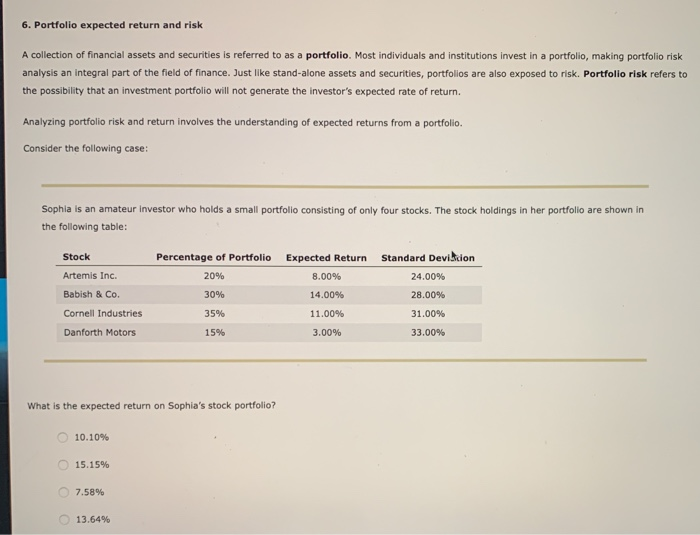6. Portfolio expected return and risk A collection of financial assets and securities is referred to as a portfolio. Most individuals and institutions invest in a portfolio, making portfolio risk analysis an integral part of the field of finance. Just like stand-alone assets and securities, portfolios are also exposed to risk. Portfolio risk refers to the possibility that an investment portfolio will not generate the investor's expected rate of return. Analyzing portfolio risk and return involves the understanding of expected...

• ### 2. 3: Risk and Rates of Return: Risk in Portfolio Context Risk and Rates of Return:...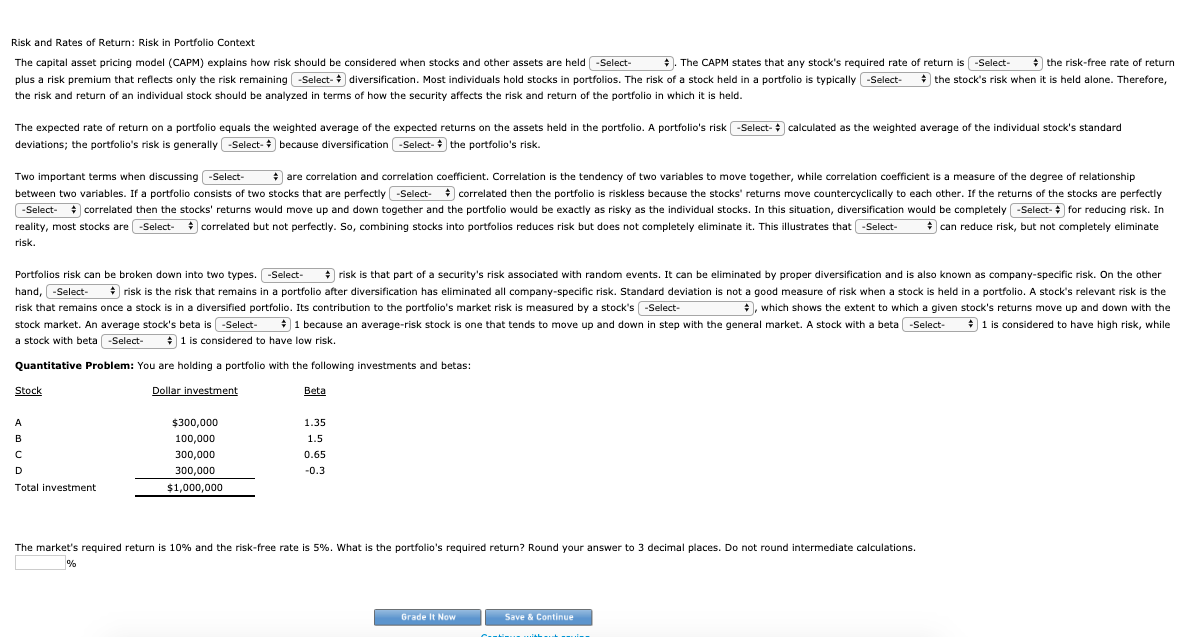2. 3: Risk and Rates of Return: Risk in Portfolio Context Risk and Rates of Return: Risk in Portfolio Context The capital asset pricing model (CAPM) explains how risk should be considered when stocks and other assets are held . The CAPM states that any stock's required rate of return is the risk-free rate of return plus a risk premium that reflects only the risk remaining diversification. Most individuals hold stocks in portfolios. The risk of a stock held in...

• ### 4. Portfolio expected return and risk Aa Aa A collection of financial assets and securities is...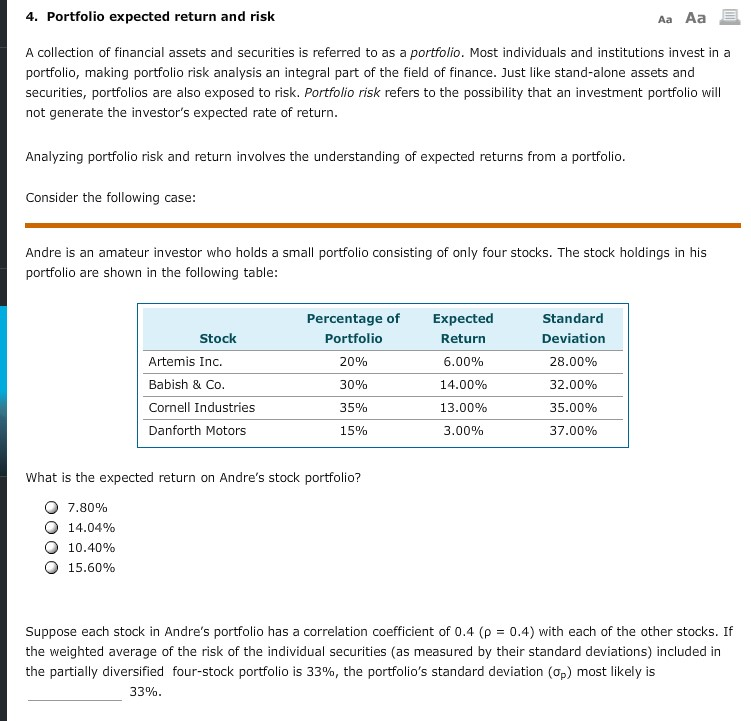4. Portfolio expected return and risk Aa Aa A collection of financial assets and securities is referred to as a portfolio. Most individuals and institutions invest in a portfolio, making portfolio risk analysis an integral part of the field of finance. Just like stand-alone assets and securities, portfolios are also exposed to risk. Portfolio risk refers to the possibility that an investment portfolio will not generate the investor's expected rate of return. Analyzing portfolio risk and return involves the understanding...

• ### Ch 02: Assignment - Risk and Return: Part 1 Term Answer Risk A Expected rate of...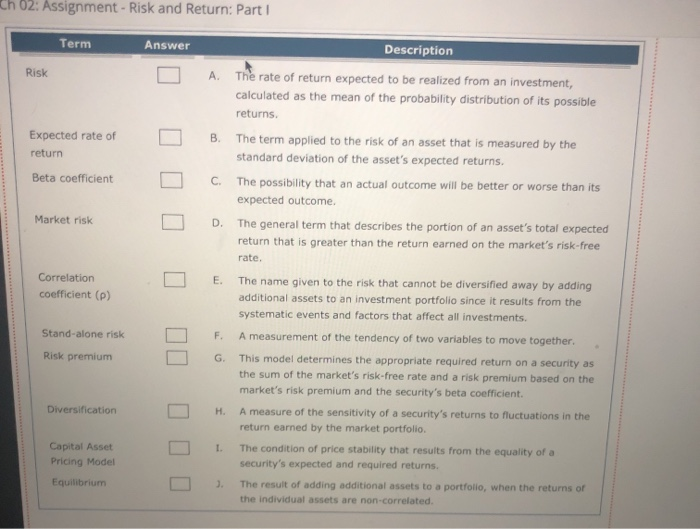Ch 02: Assignment - Risk and Return: Part 1 Term Answer Risk A Expected rate of return B Description The rate of return expected to be realized from an investment, calculated as the mean of the probability distribution of its possible returns. The term applied to the risk of an asset that is measured by the standard deviation of the asset's expected returns. The possibility that an actual outcome will be better or worse than its expected outcome The general...